## Example Questions

### Example Question #13 : Geometry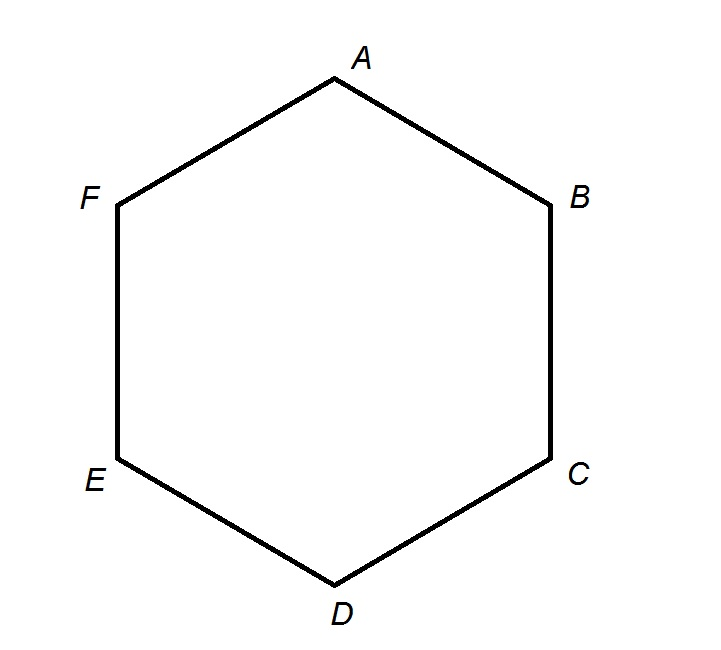Archimedes High School has an unusual track in that it is shaped like a regular hexagon, as above. Each side of the hexagon measures 264 feet.

Alvin runs at a steady speed of seven miles an hour for twelve minutes, starting at point A and working his way clockwise. When he is finished, which of the following points is he closest to?

Point C

Point B

Point D

Point F

Point E

Point E

Explanation:

Alvin runs at a rate of seven miles an hour for twelve minutes, or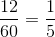hours. The distance he runs is equal to his rate multiplied by his time, so, setting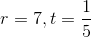in this formula: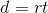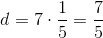miles.

One mile comprises 5,280 feet, so this is equal to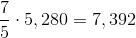feet

Since each side of the track measures 264 feet, this means that Alvin runs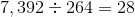sidelengths.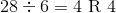,

which means that Alvin runs around the track four complete times, plus four more sides of the track. Alvin stops when he is at Point E.

### Example Question #14 : Geometry

A circle with circumference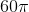is inscribed in a regular hexagon. Give the perimeter of the hexagon.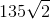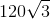None of these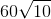Explanation:

Below is the figure referenced; note that the hexagon is divided by its diameters, and that an apothem—a perpendicular bisector from the center to one side—has been drawn.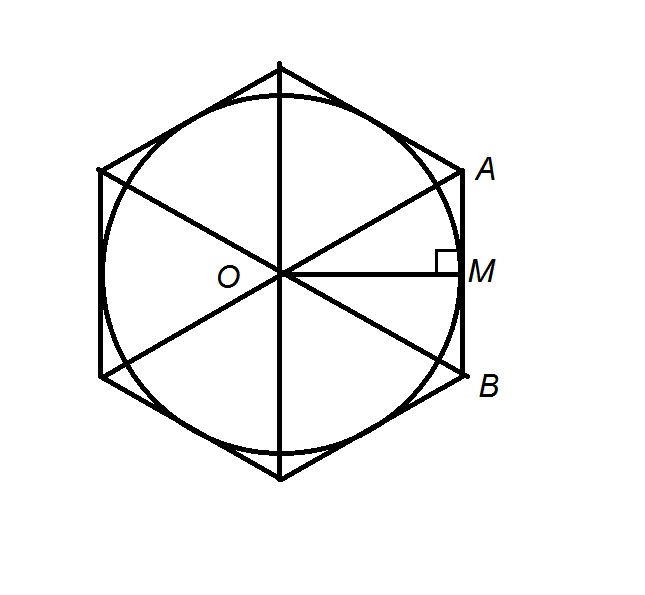The circle has circumference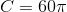; its radius, which coincides with the apothem of the hexagon,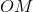is the circumference divided by: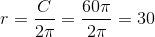The hexagon is divided into six equilateral triangles. One,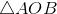, is divided by an apothem of the hexagon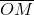- a radius of the circle - into two 30-60-90 triangles, one of which is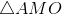. Sincehas length 30, and it is a long leg of, then short leg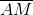has length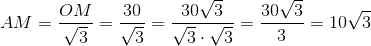is the midpoint of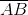, one of the six congruent sides of the hexagon, so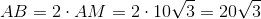;

this makes the perimeter of the hexagon six times this, or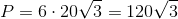.